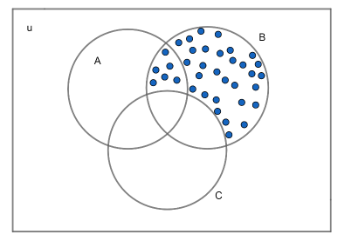QUESTION

# Let universal set $u=\left\{ 1,2,3,4,5,6,7,8,9 \right\},A=\left\{ 1,2,3,4 \right\},B=\left\{ 2,4,6,8 \right\}\ and\ C=\left\{ 3,4,5,6 \right\}$. Find $\left( B-C \right)'$.

Hint: Subtracting a set B for a set C means removing all the elements which are common in B and C from C.
Complement of a set X denoted by X’ is the set which contains all the elements that occur in the universal set (u) but not in X.

A set is a collection of well – defined objects.
We have to find $\left( B-C \right)'$.
Set $\left( B-C \right)'$ is the complement of the set $\left( B-C \right)$. For finding the complement of $\left( B-C \right)$, first we need to find the set $B-C$.
As we know $B-C$ will be set B minus all the elements which are present in both B and C.
$i.e.\ \ B-C=B-\left( B\cap C \right)$
$\left( B\cap C \right)=$ Set of the elements which are common in B and C.
Given: $B=\left\{ 2,4,6,8 \right\}\ and\ C=\left\{ 3,4,5,6 \right\}$
Elements which are common in B and C are 4 and 6.
$\Rightarrow B\cap C=\left\{ 4,6 \right\}$
By removing these elements (i.e. 4 and 6) from B, we will get $B-C$.
\begin{align} & \Rightarrow B-C=\left\{ 2,4,6,8 \right\}-\left\{ 4,6 \right\} \\ & \Rightarrow B-C=\left\{ 2,8 \right\} \\ \end{align}
We have found $\left( B-C \right)$. Now, we have to find the complement of $\left( B-C \right)$ i.e. $\left( B-C \right)'$.
We know, complement of a set X = universal set – X .
We have universal set (u) $=\left\{ 1,2,3,4,5,6,7,8,9 \right\}$
$\left( B-C \right)=\left\{ 2,8 \right\}$
And we have to find $\left( B-C \right)'$.
And we know, $\left( B-C \right)'=union-B-C$.
To find $\left( union-\left( B-C \right) \right)$, we need to find elements which are common in union and $B-C$.
\begin{align} & universal set \left( u \right)=\left\{ 1,2,3,4,5,6,7,8,9 \right\} \\ & B-C=\left\{ 2,8 \right\} \\ \end{align}
Common elements: 2 and 8
\begin{align} & \Rightarrow u-\left( B-C \right)==\left\{ 1,2,3,4,5,6,7,8,9 \right\}-\left\{ 2,8 \right\} \\ & \Rightarrow \left( B-C \right)'=\left\{ 1,3,5,6,7,9 \right\} \\ \end{align}
Hence, the required $\left( B-C \right)'=\left\{ 1,3,5,6,7,9 \right\}$

Note: We can also find $\left( B-C \right)'$ by using Venn diagrams.We will get the dotted area if we subtract B from ‘C’ and if we will subtract this dotted area from the union, we will get the entire un-dotted area.